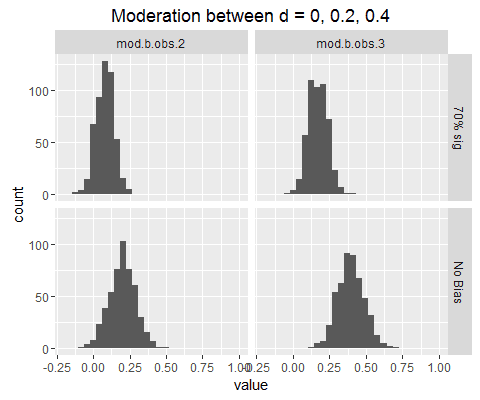## Monday, February 27, 2017

### Publication bias can hide your moderators

It is a common goal of meta-analysis to provide not only an overall average effect size, but also to test for moderators that cause the effect size to become larger or smaller. For example, researchers who study the effects of violent media would like to know who is most at risk for adverse effects. Researchers who study psychotherapy would like to recommend a particular therapy as being most helpful.

However, meta-analysis does not often generate these insights. For example, research has not found that violent-media effects are larger for children than for adults (Anderson et al. 2010). Similarly, it is often reported that all therapies are roughly equally effective (the "dodo bird verdict," Luborsky, Singer, & Luborsky, 1975; Wampold et al., 1997)."Everybody has won, and all must have prizes. At least, that's what it looks like if you only look at what got published."

It seems to me that publication bias may obscure such patterns of moderation. Publication bias introduces a “small-study effect” in which the observed effect size is highly dependent on the sample size. Large-sample studies can reach statistical significance with smaller effect sizes. Small-sample studies can only reach statistical significance by reporting enormous effect sizes. The observed effect sizes gathered in meta-analysis, therefore, may be more a function of the sample size than they are a function of theoretically-important moderators such as age group or treatment type.

In this simulation, I compare the statistical power of meta-analysis to detect moderators when there is, or when there is not, publication bias.

### Method

Simulations cover 4 scenarios in a 2 (Effects: large or medium) × 2 (Pub bias: absent or present) design.

When effect sizes were large, the true effects were δ = 0 in the first population, δ = 0.3 in the second population, and δ = 0.6 in the third population. When effect sizes were medium, the true effects were δ = 0 in the first population, δ = 0.2 in the second population, and δ = 0.4 in the third population. Thus, each scenario represents one group with no effect, a group with a medium-small effect, and a group with an effect twice as large.

When studies were simulated without publication bias, twenty studies were conducted on each population, and all were reported. When studies were simulated with publication bias, studies were simulated, then published and/or file-drawered until at least 70% of the published effects were statistically significant. When results were not statistically significant and were file-drawered, further studies were simulated until 20 statistically significant results were obtained. This keeps the number of studies k constant at 20, which prevents confounding the influence of publication bias with the influence of fewer observed studies.

For each condition, I report the observed effect size for each group, the statistical power of the test for moderators, and the statistical power of the Egger test for publication bias. I simulated 500 meta-analyses within each condition in order to obtain stable estimates.

### Results

#### Large effects.

Without publication bias:
• In 100% of the metas, the difference between δ = 0 and δ = 0.6 was detected.
• In 92% of the metas, the difference between δ = 0 and δ = 0.3 was detected.
• In only 4.2% of cases was the δ = 0 group mistaken as having a significant effect.
• Effect sizes within each group were accurately estimated (in the long run) as δ = 0, 0.3, and 0.6.

With publication bias:
• Only 15% of the metas were able to tell the difference between δ = 0 and δ = 0.3.
• 91% of meta-analyses were able to tell the difference between δ = 0 and δ = 0.6.
• 100% of the metas mistook the δ = 0 group as having a significant effect.
• Effect sizes within each group were overestimated: d = .45, .58, and .73 instead of 0, 0.3, and 0.6.
Here's a plot of the moderator parameters across the 500 simulations without bias (bottom) and with bias (top).Moderator values are dramatically underestimated in context of publication bias.

#### Medium effects.

Without publication bias:
• 99% of metas detected the difference between δ = 0 and δ = 0.4.
• 60% of metas detected the difference between δ = 0 and δ = 0.2.
• The Type I error rate in the δ = 0 group was 5.6%.
• In the long run, effect sizes within each group were accurately recovered as d = 0, 0.2, and 0.4.

With publication bias:
• Only 35% were able to detect the difference between δ = 0 and δ = 0.4.
• Only 2.2% of the meta-analyses were able to detect the difference between δ = 0 and δ = 0.2,
• 100% of meta-analyses mistook the δ = 0 group as reflecting a significant effect.
• Effect sizes within each group were overestimated: d = .46, .53, and .62 instead of δ = 0, 0.2, and 0.4.
Here's a plot of the moderator parameters across the 500 simulations without bias (bottom) and with bias (top).Again, pub bias causes parameter estimates of the moderator to be biased downwards.

### Conclusion:

Publication bias can hurt statistical power for your moderators.  Obvious differences such as that between d = 0 and d = 0.6 may retain decent power, but power will fall dramatically for more modest differences such as that between d = 0 and d = 0.4. Meta-regression may be stymied by publication bias.